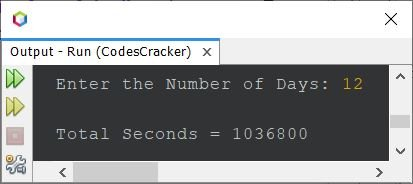# Java Program to Convert Days to Seconds

This article contains a program in Java to convert a given number of days into seconds. There are 86400 seconds in a day.

The question is: write a Java program to convert days into seconds. The days must be received by the user at runtime. The program given below is an answer to this question.

```import java.util.Scanner;

public class CodesCracker
{
public static void main(String[] args)
{
int noOfDays, noOfSeconds;
Scanner s = new Scanner(System.in);

System.out.print("Enter the Number of Days: ");
noOfDays = s.nextInt();

noOfSeconds = noOfDays*86400;
System.out.println("\nTotal Seconds = " +noOfSeconds);
}
}```

Here is its sample run with user input of 12 as the number of days to convert, find, and print the total number of seconds passed in the given 12 days:The user is prompted to enter the number of days before this Java program calculates the total number of seconds in those days. To enable keyboard data entry, it begins by importing the Scanner class from the java.util package. The next two integer variables, noOfDays and noOfSeconds, are then declared.

The program prompts the user to enter the number of days using the System.out.print() and s.nextInt() methods of the Scanner class. It then uses the formula noOfSeconds = noOfDays*86400 to calculate the total number of seconds in noOfDays.

Finally, it uses the System.out.println() method to display the outcome. The total number of seconds is shown on the output.

This type of Java program comes under the category of simplest program, as here we only need to receive the input from the user and then multiply by 86400. The result will be the total number of seconds in the given number of days. So we only need to print the result value on the output. That's it.

To make the above program shorter, replace all the statements present inside the main() method with these statements:

```Scanner s = new Scanner(System.in);
System.out.print("Enter the Number of Days: ");
int noOfDays = s.nextInt();
System.out.println("\nTotal Seconds = " +noOfDays*86400);```

The output will be exactly the same as in the previous program.

Java Online Test

« Previous Program Next Program »